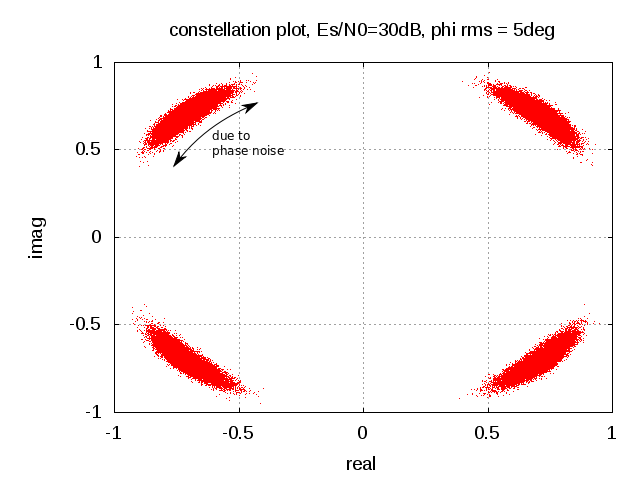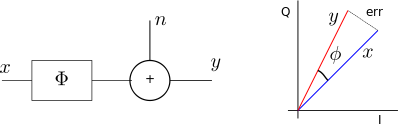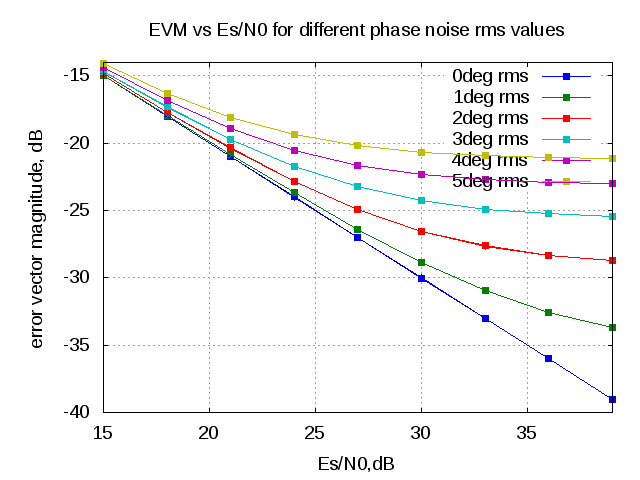# EVM with phase noiseThe previous post on phase noise discussed about finding the root mean square phase noise for a given phase noise profile. In this post let us discuss about the impact of phase noise on the error vector magnitude (evm) of a transmit symbol.

## Error Vector Magnitude due to constant phase offset

Consider a system model introducing a constant phase offset and thermal noise  as shown in the figure.Figure : System model with phase noise and thermal noise

,

where

is the phase distortion in radians,

is the transmit symbol and

is the contribution due to thermal noise

Expanding into real and imaginary components,

.

Representing in matrix algebra,

The power of the error vector is,

.

Finding the average error over many realizations,

.

The individual terms can be simplified as,

i)

as .

ii)

as and are uncorrelated.

iii) .

iv) , the variance of the noise.

Applying (i), (ii) , (iii) and (iv), the error term simplifies to

.

## EVM due to random phase offset

The above equation derives the evm when the system is affected by a constant phase offset .   Assume that the phase is Gaussian distributed with zero mean and variance  radians^2 having a probability density function as,

.

The conditional error power for a given phase  is,

.

Computing the average over all realization of phase,

.

The integral term is,

(Note : proof will be discussed in another post)

Then the error vector power is,

and the error vector magnitude is,

Using Taylor series, and assuming that the  is small,

,Figure : Example constellation plot (Es/N0=30dB, =5 degrees)

## Matlab/Octave example

Attached script computes the evm of a QPSK modulated symbol versus Es/N0 for different values of rms phase noise.

% Script for simulating the error vector magnitude (evm) of a QPSK
% modulated symbol affected by phase noise and thermal noise
% ----------------------------------------------------------

clear;close all;
N = 10^5 % number of bits or symbols

Es_N0_dB = [15:3:40]; % multiple Eb/N0 values
phi_rms_deg_vec = [0:1:5];

for ii = 1:length(Es_N0_dB)
for jj = 1:length(phi_rms_deg_vec)
% Transmitter
ip_re = rand(1,N)>0.5; % generating 0,1 with equal probability
ip_im = rand(1,N)>0.5; % generating 0,1 with equal probability
s = 1/sqrt(2)*(2*ip_re-1 + j*(2*ip_im-1)); % QPSK modulation

n = 1/sqrt(2)*[randn(1,N) + j*randn(1,N)]; % thermal noise
phi = phi_rms_deg_vec(jj)*(pi/180)*randn(1,N); % phase noise

y = s.*exp(j*phi) + 10^(-Es_N0_dB(ii)/20)*n;

% error vector
error_vec = (y-s);

evm(ii,jj)  = error_vec*error_vec';
theory_evm(ii,jj) =  10^(-Es_N0_dB(ii)/10) + 2 - 2*exp(-(phi_rms_deg_vec(jj)*pi/180).^2/2);
end
end

figure;
plot(Es_N0_dB,10*log10((evm/N)),'s-');
hold on;grid on
plot(Es_N0_dB,10*log10(theory_evm),'+-');
xlabel('Es/N0,dB');
ylabel('error vector magnitude, dB');
title('EVM vs Es/N0 for different phase noise rms values');
legend('0deg rms','1deg rms','2deg rms','3deg rms','4deg rms','5deg rms')Figure : EVM vs Es/N0 for different values of rms phase noise

## Summary

As a quick rule of thumb, for a system  with rms phase noise of 1 degree, the evm due to phase noise alone is -35.16dB and rises by 6dB per octave (or 20dB per decade).

The phase noise profile used in this simulations assumes a Gaussian distributed flat spectrum, which is not the case in typical phase noise profiles. The EVM with a classical phase noise profile will be discussed in another post.

## References

 Georgiadis, A.; , “Gain, phase imbalance, and phase noise effects on error vector magnitude,” Vehicular Technology, IEEE Transactions on , vol.53, no.2, pp. 443- 449, March 2004
doi: 10.1109/TVT.2004.823477
URL: http://ieeexplore.ieee.org/stamp/stamp.jsp?tp=&arnumber=1275708&isnumber=28551

## 9 thoughts on “EVM with phase noise”

1.Koushik says:

Hi Krishna,
Thank you for hosting this site – you are doing some great work here! I know this blog entry is old, and I hope you see my message, I have couple of questions –

1. The EVM calculations you derive – what assumptions do you do on the channel? will you see a 3dB degradation in the EVM if you do not estimate the channel?
Ex: Say, I have a transmitter that is only phase noise limited (DoubleSideBand rms noise is 1degree), and I measure the EVM through Litepoint (as I would to pass IEEE specification), will I measure -35.16dB or -32.16dB EVM?
2. Is the EVM calculations you perform hold good for OFDM systems? The reason I ask is, I have seen EVM being a function of phase noise offset, and hence the PSD of noise system affects the EVM (perhaps your derivations holds good for this scenario too, until you make the assumption that the phase noise is Gaussian distribution)

Thank you
Koushik

1.Krishna Sankar says:

@Koushik: Thanks. My replies:
1. In the EVM calculation, as we can see from the equations, the channel is assumed to be unity (AWGN) and known apriori. The channel is not playing a role in the above equations.
In the case where channel is not known and we use a noisy estimate of channel, expecting a 3dB drop in the expected EVM (due to two independent noise sources).

2. In the above post, am assuming a gaussian distributed white phase noise. This relation should hold good in general for OFDM too.
However as you said, with OFDM the preference will be for a phase noise distribution having more lower frequency components (lesser than the subcarrier spacing) to avoid inter subcarrier interference.

Helps? 🙂

2.priya says:

Hello Mr.Krishna,

I need some help on my project.How the Probability of bit error changes(for each modulation) when there is a phase error(phase noise)(let it be tetha).let it be, 2 cases of phase errors be possible,(oscillator phase noise(Tx side),PLL untracked phase noise(rx side).and my assumption is both are gaussian,and their variance are sum of 2 individual gaussian process.I understood that the now the Probabilty of error is
the product of probability of bit error conditioned on phase error(tetha) and Probability distribution of phase error,

my question here is i know the Pdf of phase error is gaussian.and how can i calculate the Probability of error conditioned on phase error?(P(E|tetha)??
help me to find out this,send me ur Email id.to discuss abt my project.

regards,
priya Neelakandan

1.Krishna Sankar says:

Georgiadis, A.; , “Gain, phase imbalance, and phase noise effects on error vector magnitude,” Vehicular Technology, IEEE Transactions on , vol.53, no.2, pp. 443- 449, March 2004

3.farah says:

hi mr krishna,

email subject is SOS..

1.Krishna Sankar says:

@farah: emailed you some pointers

4.Basel Nabulsi says:

Hi Krishna,

Noting the noise for oversampling, what is the modified formula for oversampling?
Let’s say I have bpsk with a given data rate. If I oversample the symbol N times how would that modify the SNR?
(Eb/N0)new = (Eb/N0)old – 10log10(N)

1.Krishna Sankar says:

@Basel: You are right. When we oversample, the noise gets spread over a wider bandwidth. Assuming that the noise outside the desired signal bandwidth gets filtered out, the inband SNR improves as stated your formula i.e for every doubling, the SNR improves by 3dB